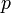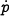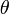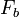# Balance systems

The figure to the right show a schematic diagram of a simple balance system. To model this system, we choose state variables that represent the position and velocity of the base of the system,and, and the angle and angular rate of the structure above the base,and. We letrepresent the force applied at the base of the system, assumed to be in the horizontal direction (aligned with), and choose the position and angle of the system as outputs. With this set of definitions, the dynamics of the system can be computed using Newtonian mechanics and has the form: